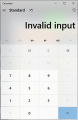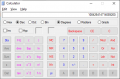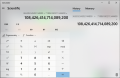# 64 bit numbers addition bug in Windows and Excel

#### cmartinez

Joined Jan 17, 2007
7,750
I wrote a pic assembly routine to add very large numbers (64 bit, that is eight-byte numbers) And after some testing, I found a difference between my results and the result reported by the windows calculator. Even Excel yields an erroneous result!

036530234502744800 +
071896180211344400

Excel reports a value of:
108426414714089000

As can very easily be seen, manually adding the first three digits DOES NOT match the answer reported by Excel. I've also detected the same problem when using the Windows Calculator. And then I went online to a few sites and discovered that they too report errors when dealing with numbers of this magnitude.

In fact, take a look at the following addition as shown by Excel:

00000000000000001
00000000000002816
00000000001638400
00000000050331648
00000060129542144
00219902325555200
36310271995674600
-----------------------
36530234502744800

Simply manually adding THE FIRST COLUMN DIGITS (which yields a value of 19) does not correspond to the result as it should be! What gives? Would this have to do with internal floating point operations?

#### JohnInTX

Joined Jun 26, 2012
4,669

#### DickCappels

Joined Aug 21, 2008
8,838
\$036530234502744800 no wonder my budget calculations don't work out as planned!

#### BobTPH

Joined Jun 5, 2013
5,259
Never heard of anyone calculating their budget in picocents before!

Bob

•jblank

#### MrChips

Joined Oct 2, 2009
26,530
•strantor and cmartinez

#### cmartinez

Joined Jan 17, 2007
7,750
Don't use floating point arithmetic.

The Perils of Floating Point
I try to avoid it my designs like the plague. And when it's become necessary to use it, I do a careful analysis as to the best order in which to perform calculations so as to minimize error accumulation (or "error travel", as I like to call it).

•nsaspook

#### nsaspook

Joined Aug 27, 2009
9,993
Don't use floating point arithmetic.

The Perils of Floating Point
Don't use floating point arithmetic unnecessarily, even in FORTRAN."While some of the "perils" can be avoided, many just need to be understood and accepted"

•cmartinez

#### MrChips

Joined Oct 2, 2009
26,530
My favorite hack is how to write code to convert from °C to °F and vice versa without having to resort to floating point math.

#### ErnieM

Joined Apr 24, 2011
8,315
Don't use floating point arithmetic.
I remember one day coming into work to find my idiot boss in near tears at his desk. His dilemma was our new embedded project for a capacitive level sensor and he had noticed to compute the height we needed to do some math in the form A/B * C, and since B >> A this NECESSITATED the use of floating point math, a bigger processor, paying for a compiler....

I just pointed out that if we first performed A * C, and then divide that result by B, at no time was our answer <1.

He spent the next several hours repeating his analysis, but this is the man who's reaction to an op amp circuit (one amp plus some resistors) I showed him was "but is that linear?"

•cmartinez

#### nsaspook

Joined Aug 27, 2009
9,993
Last edited:
•cmartinez

#### MrChips

Joined Oct 2, 2009
26,530
No, by using signed binary integers. I had intended to write a blog on this. Maybe one of this days I will.

Let us be modest and begin with °C with 0.1°C resolution.
8-bit integers will provide a limited range of 0-25.5°C.
We have to use 16-bit signed integers which will give us a range of -3276.8 to +3276.7.
This is more than adequate. In previous projects I have used integers scaled by 40. The range becomes -819 to +819. This allows for rounding errors in calculations.

Now let us look at a specific example of °C to °F conversion. Let us use a fixed point scale of 10 to keep it simple.

The conversion is:
°F = (°C x 9 / 5) + 32

°F and °C values are scaled by a factor of 10. The display routine will take care of the fixed point representation by inserting a decimal point before the last digit.

The conversion becomes:
°F = (°C x 9 / 5) + 320

The goal is to reduce any multiplication and division to a simple multiplication.
Here I will scale my values by 8 bits (i.e. a factor of 256).

(9 / 5) becomes
(9 / 5) x (256 / 256) = (9 x 256 / 5) /256 = 460.8 / 256 ≅ 461 / 256

Thus (9/5) is computed as (461/256).

Let us use the example of 50.0°C to 122.0°F.

C = 500
F = (C x 9/5) + 320 = (C x 461 / 256) + 320 = (230500 / 256) + 320 = 900 + 320 = 1220

Hence the result is °F = 122.0

Note that 16 x 16 multiply will give 32-bit result.
Division by 256 is simply dropping the least significant byte of the 4-byte result.

•cmartinez

#### DickCappels

Joined Aug 21, 2008
8,838
Thank you for the explanations. Now I understand why, on a (different) forum I was beat up for asking how to represent variables as floating point numbers in C. I ended up using double precision which was a lot more work that I hoped to have to do, but did not know why it was a good idea until now.

#### panic mode

Joined Oct 10, 2011
2,175
Win10 64-bit calculator does not even like the first numberWinXP calculator (a 32-bit app) has no problem with this and returns correct resultClearly some lazy programmers had preference to make things look flashy rather than making their products perform.
Ok let's switch Win10 calc to Scientific mode. Ok, that is better:•atferrari and cmartinez

#### panic mode

Joined Oct 10, 2011
2,175
i was not surprised with this issue at all as i have seen it plenty of times.
the only surprise was with Excel which i did not test previously in this respect.
so i was expecting that formatting cell to currency would use calculations based on Currency data type. apparently not so....
in Excel currency just slaps some string format to result but calculations are still done in 64-bit. well that sucks...

#### cmartinez

Joined Jan 17, 2007
7,750
No, by using signed binary integers. I had intended to write a blog on this. Maybe one of this days I will.

Let us be modest and begin with °C with 0.1°C resolution.
8-bit integers will provide a limited range of 0-25.5°C.
We have to use 16-bit signed integers which will give us a range of -3276.8 to +3276.7.
This is more than adequate. In previous projects I have used integers scaled by 40. The range becomes -819 to +819. This allows for rounding errors in calculations.

Now let us look at a specific example of °C to °F conversion. Let us use a fixed point scale of 10 to keep it simple.

The conversion is:
°F = (°C x 9 / 5) + 32

°F and °C values are scaled by a factor of 10. The display routine will take care of the fixed point representation by inserting a decimal point before the last digit.

The conversion becomes:
°F = (°C x 9 / 5) + 320

The goal is to reduce any multiplication and division to a simple multiplication.
Here I will scale my values by 8 bits (i.e. a factor of 256).

(9 / 5) becomes
(9 / 5) x (256 / 256) = (9 x 256 / 5) /256 = 460.8 / 256 ≅ 461 / 256

Thus (9/5) is computed as (461/256).

Let us use the example of 50.0°C to 122.0°F.

C = 500
F = (C x 9/5) + 320 = (C x 461 / 256) + 320 = (230500 / 256) + 320 = 900 + 320 = 1220

Hence the result is °F = 122.0

Note that 16 x 16 multiply will give 32-bit result.
Division by 256 is simply dropping the least significant byte of the 4-byte result.
Chips, I just wanted to say THANK YOU for this small explanation of yours. I have to convert from bar to kg/cm² and my life's become the hell of a lot easier thanks to your hack.

Cheers!

•nsaspook and MrChips

#### MrChips

Joined Oct 2, 2009
26,530
Almost any number can be represented as A/B.
Make B a power of 2 so that division by B can be implemented as a right-shift operation.

#### ErnieM

Joined Apr 24, 2011
8,315